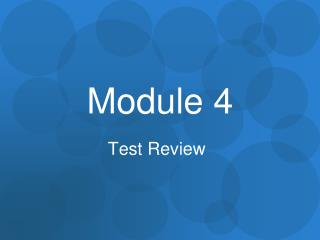DownloadDownload PresentationModule 4

# Module 4

Télécharger la présentation## Module 4

- - - - - - - - - - - - - - - - - - - - - - - - - - - E N D - - - - - - - - - - - - - - - - - - - - - - - - - - -
##### Presentation Transcript

1. Module 4 Test Review

2. Adding and Subtracting Fractions ****Don’t forget to find the LCD when you add and subtract fractions

3. Let’s Practice

4. Adding and Subtracting Mixed Numbers *** EASIEST way to add and subtract mixed fractions : Turning them into Improper fractions FIRST! ****Don’t forget to find the LCD when you add and subtract fractions

5. Let’s Practice

6. Multiplying Fractions Is as easy as your ABC’s! * = Hint… if you have mixed numbers, turn them into improper fractions and then just multiply the same way

7. Let’s Practice

8. Dividing Fractions • Keep the first fraction alone • Switch the sign to multiplication • Flip the second fraction K S F ÷ = * Is now Hint… if you have mixed numbers, turn them into improper fractions and then just divide the same way

9. Let’s Practice

10. Please go to your assessment tab and take the module 4 test